Mean Power is not RMS Power for a sinusoidal voltage.
Dr. David Kirkby, G8WRB. 26/3/2005

This was done in an attempt to settle an argument about RMS power, that appeared on the  'amps' mailing list in March 2005. Not that arguments every seem to get settled there (especially the parasitic oscillations), but this might be a bit more scientific.

Definition of RMS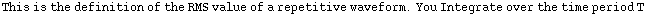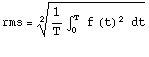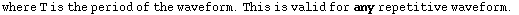Definition of mean value of a continuous repetitive waveform
mean=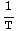f(t)  dt

Finding the RMS value of a sine wave.

First find the RMS value of a sine wave, with a peak of 1.
The period T of a sine wave is 360° or, expressed more mathematically, it is 2π radians.

Here's a plot of a sine wave over 2π radians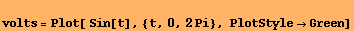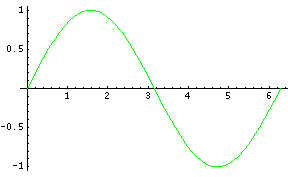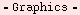You can see, that is a complete cycle.

Now the rms value of this will be found. We define the voltage as sin(t).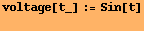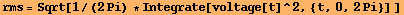Convert the above to a floating point number.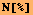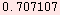So no great surprise there.

Finding the mean of a sine wave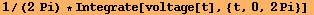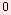So the mean value of voltage on a sine wave is 0, the rms is 0.707 times the peak.

Instantaneous power.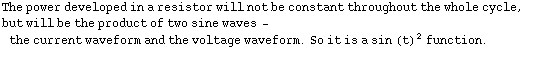We will define the current to be sinusoidal too, just like for a voltage.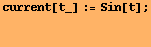Now here's a plot of the instantaneous power.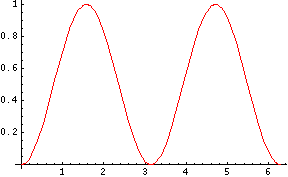Note, that is not the same as a rectified sine wave, which is found by plotting the absolute value of a sine wave, as below.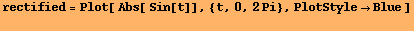Finding the RMS value of power.

To find the rms value of the power, we note that power is the product of the voltage and current. and go back to our definition of RMS
rms=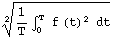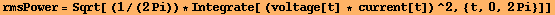Now to find the numerical value of that.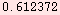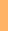Finally, here is a graph showing the voltage (green), the power (red) and that of a rectified sine wave (blue)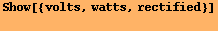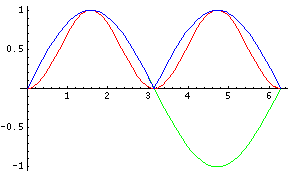Note, since the power waveform actually repeats every π radians, we could integrate over π rather than 2π in the case of the power, but not in the case of the voltage or current, as they have a period of 2π radians.

Conclusions

The RMS vale of a sine wave is 0.707 x the peak value. Hence the mean voltage of a sine wave is 0.
The instantaneous power, which is the product of voltage and current, looks similar(ish) to a rectified sine wave, but it is a sine wave squared. The RMS value of the power waveform can be found, and the answer is 0.612372 times the peak of the voltage waveform. The mean value of power of is 0.5*Vpeak*Ipeak, or Vrms*Irms.

The most important conclusion is that the mean power and rms power are not the same for a sinusoidal voltage applied to a resistor. The RMS power is larger by a factor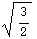= 1.224744871391589

I'm sure this will never settle an argument on 'amps', but I tried.

73 de G8WRB. 26/3/2005

 Created by Mathematica  (March 26, 2005)